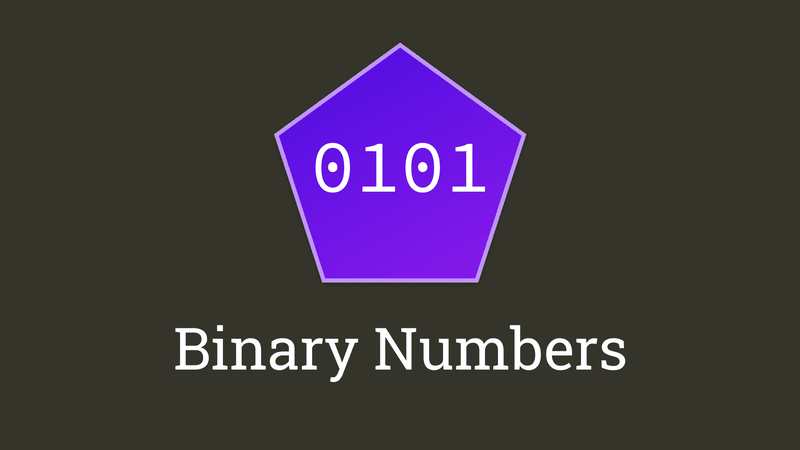# Making Sense Of Binary Numbers

Working with binary numbers doesn't have to be hard, even though most people are convinced that it is. Here's a understandable guide for everyone!

Created by Maximilian Schwarzmüller

Binary numbers are quite common in development - no matter if you're working as a web developer, mobile app developer or any other kind of developer.

Whilst you might not be working with binary numbers too often, you are actually using them implicitly all the time. And if you ever encountered some programming language quirks like rounding errors with certain numbers, you definitely got in touch with binary numbers - maybe without even noticing it.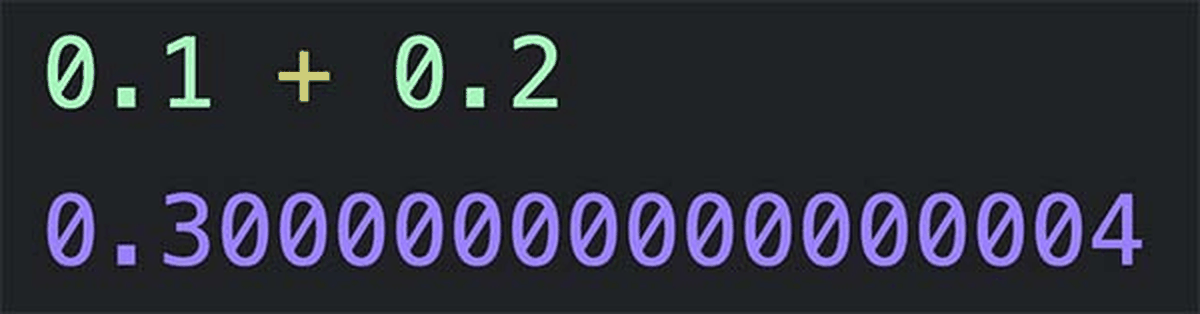#

## What Is A Numeral System?

Numeral systems like the decimal system (our "main system" that we use as humans) or the binary system (the "main system" used internally by computers) are simply "collections of rules" that help us express numbers. That's the essential idea behind those systems.

For example, in the decimal system, we have the "standalone" numbers `0` to `9`. That's our main rule: These nine numbers are the numbers that we may work with. All other numbers have to be formed with help of these numbers.

For example, the number `18` is the combination of `1` and `8`.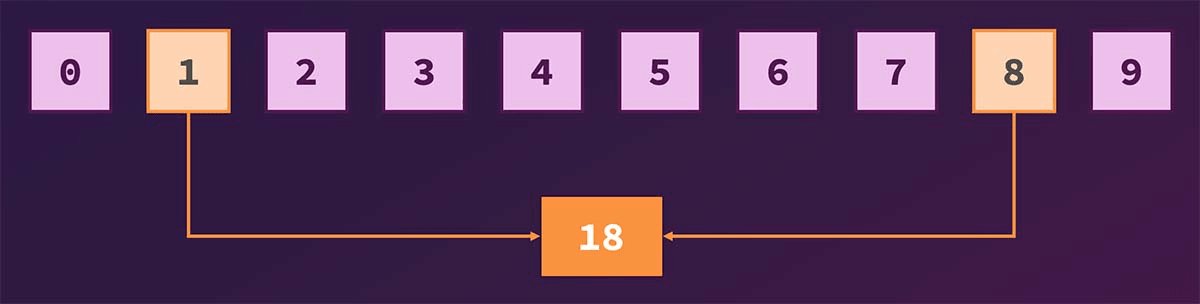But it's actually not just about combining digits into numbers. There are clear rules that define how numbers bigger than 9 (the highest "standalone number" available) can be derived. We'll take a look at those rules later!

For us humans, it's super intuitive and logical to think in the decimal system - that's why it can be difficult to switch into another system. But this article will help you make that switch more easily!

#

## Why Do We Have Numeral Systems?

You can think of numeral systems as equivalents to dictionaries - numeral systems are dictionaries for numbers:

• The English dictionary (for words) contains a list of words that you may use to form sentences.

• The decimal numeral system contains the numbers that you may use to do math and form more numbers.

Makes sense?

We have numeral systems so that we have a "language" that we can speak for doing math - if people would start inventing their own numbers or mixing numbers from different systems, we wouldn't be able to derive proper results.

#

## You Might Not Really Understand The Decimal System

Chances are high that you know how to work with the decimal numeral system (since you are doing it every day) but you actually don't fully understand why you're using it like this!

So here's a brief refresher that will help with understanding numeral systems in general.

In the decimal system, you have nine "standalone numbers" at your disposal: `0` to `9`.

If you want to express or use the number `5`, you simply use the number `5`. It's available just like that!

But what if you need the number `15`? You only have the numbers `0` to `9` after all.

What we do intuitively (and probably without really knowing it) in such cases is the following:

1. We take the biggest number available (`9`) and see that it's too low (it's smaller than `15`)

• Hence we restart at `0` but we add a `1` on the left because we exceeded our range (`0 - 9`) once

• We now count up again and reach `5` without exceeding our range again

• Together with the `1` which we memorized, this yields `15`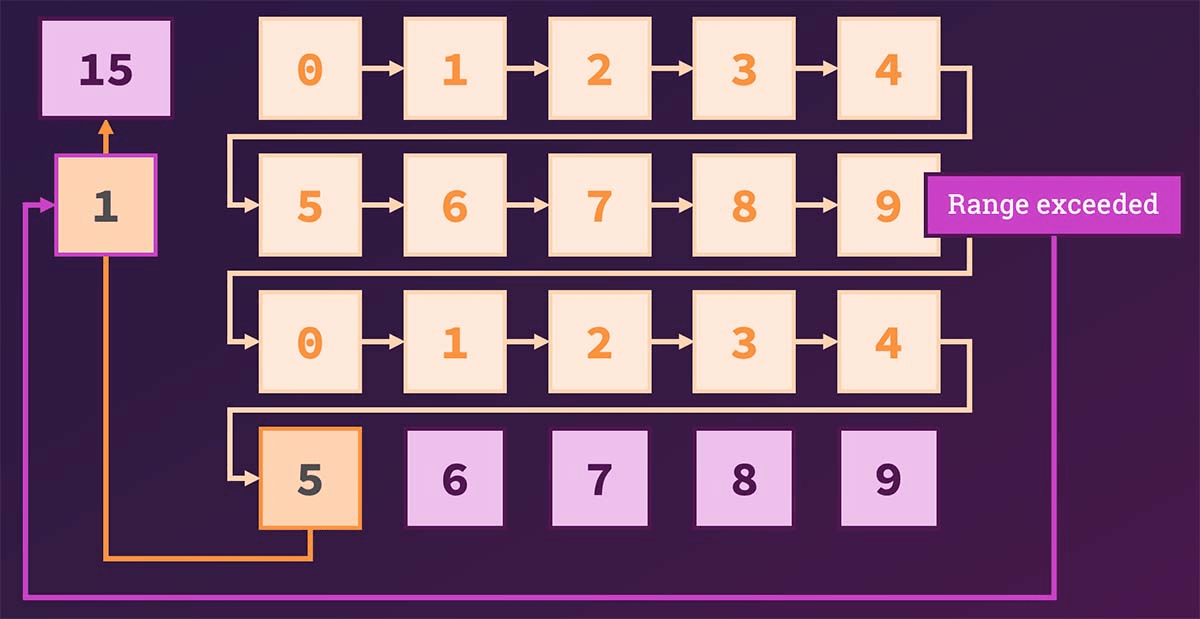This approach might sound a bit weird but that is how numbers are derived. This is what allows us to do any math because we, for example, know that `15` is greater than `9` (we know this because we had to count above `9` to reach `15`).

If we would want to work with the number `23`, we would derive it by exceeding our range (`0 - 9`) twice. Hence we add a `2` (=> "twice") on the left and then combine that with `3` which is now in our range again.

More formally, all numbers can be expressed like this:

`1 = 1 * 10⁰ - exceeded the range 0 times8 = 8 * 10⁰10 = 1 * 10¹ + 0 * 10⁰ - exceeded the range 1 time39 = 3 * 10¹ + 9 * 10⁰895 = 8 * 10² + 9 * 10¹ + 5 * 10⁰12.19 = 1 * 10¹ + 2 * 10⁰ + 1 * 10⁻¹ + 9 * 10⁻²`

(Keep in mind that

Do you see the pattern?

You express numbers as multiples of `base 10` to the power of incrementing numbers (`0`, `1`, `2` in the example above). I like to refer to the exponent as "how often did we exceed our range of allowed numbers?" (i.e. for `10⁰`, we did not exceed it at all, for `10¹`, we exceeded it once).

We use `base 10`, because we're talking about the decimal numeral system here and `10` is the first number that we can't express. That's how numeral systems work - for the binary system (covered below), we work with `base 2`.

Of course, as mentiond before, you probably don't think about our numbers like this. Because you're intuitively working with them in the correct way. The decimal system also makes a lot of sense to us humans, because we have ten fingers.

But understanding this theory will help with understanding the binary numeral system - which is the system we'll explore next!

#

## What Is The Binary Numeral System?

The binary numeral system follows the same idea as the decimal numeral system: It's simply a "dictionary" of available numbers.

The key difference is that we have way less numbers available: Only the numbers `0` and `1`.

Computers typically use the binary system internally, because they know two states: On and Off. This can be represented perfectly in the binary system (`0` = Off, `1` = On).

In the binary system, we therefore have to express all numbers with these two numbers. But since we humans alwys think in the decimal system, we may ask ourselves questions like: "What would a `3` look like in the binary system?".

If you think about it, the question is rather strange because you're asking about a value from the decimal system (`3`). It's a bit like asking: "What is `f` like in the decimal system?". `f` is a number from the hexadecimal system - a system that knows numbers from `0` to `f` (where `f` stands for `15`).

In the binary system, there simply is no `3` - just as there is no `f` in the decimal system.

Instead, just as mentioned before for the decimal numeral system, we express numbers with help of `0` and `1` and the question "how often do we exceed our range of allowed numbers?".

For example, the number `10` in the binary numeral system is a number where we "exceeded" our range once. Just as with `10` in the decimal system.

But `10` in the binary system is actually the number `2` in the decimal system (if you think about it, it should make sense: `2` is greater than `1`, which is the biggest number we have in the binary system - hence we exceeded the range).

Why?

That's what we'll explore next!

#

## Converting Decimal Numbers To Binary Numbers

Since we only have two numbers available, all numbers have to be expressed with these two values.

Here are some conversion examples (decimal => binary):

`0 = 01 = 12 = 103 = 114 = 10020 = 10100`

What's the rule here? How does this work?

Remember that we expressed `10` like this in the decimal system:

`10 = 1 * 10¹ + 0 * 10⁰`

The `0 * 10⁰` part might look redundant because `1 * 10¹` already results in `10`, but by always writing down all the summands until we reach `10⁰`, we can actually take the multipliers (`1` and `0`) and combine them to the final number: `10` (i.e. we're concatenating the two numbers). And that is how we do actually "build" our numbers in the decimal system.

We can do the same thing for the binary numeral system (just with `base 2` instead of `base 10` of course)!

`0 = 0 * 2⁰ => 01 = 1 * 2⁰ => 12 = 1 * 2¹ + 0 * 2⁰ => 103 = 1 * 2¹ + 1 * 2⁰ => 11`

If we write it like this, and we use the same approach as described above for the decimal system (=> taking the multipliers and concatenating them), it's easy to convert `3` to `11` in the binary system since `3` can be written as `1 * 2¹ + 1 * 2⁰` if we have to use `base 2` and we write down all multipliers until we reach `2⁰`.

#

## Converting Bigger Numbers

This approach also works for bigger numbers:

`113 = 1 * 2⁶ + 1 * 2⁵ + 1 * 2⁴ + 0 * 2³ + 0 * 2² + 0 * 2¹ + 1 * 2⁰`

Hence `113` from the decimal system is written as `1110001` in the binary system.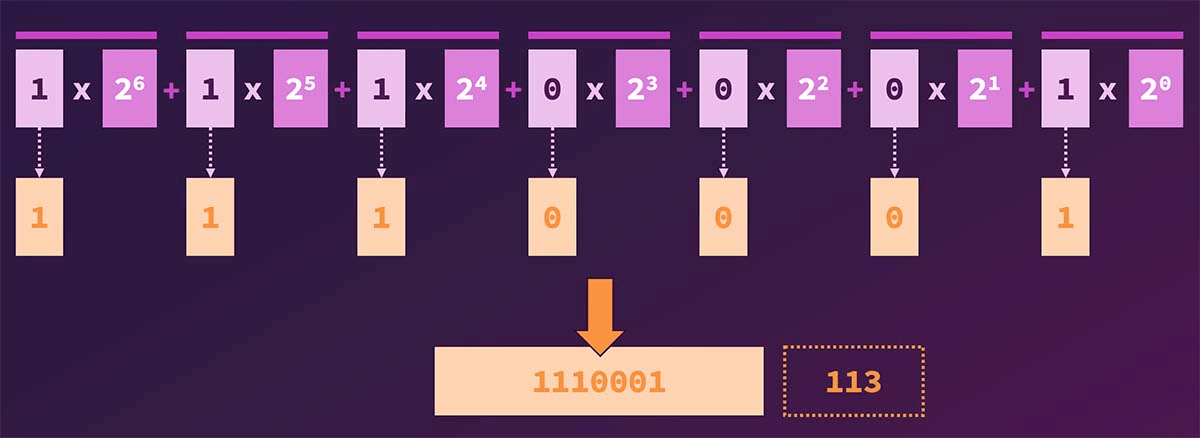For big numbers like this, it's easiest if you simply keep dividing the number by `2` until you reach a number equal to `1` - this helps you find out how many multipliers you'll need:

`113 / 2 = 56 (remainder: 1 because 113 = 112 + 1 where 112 / 2 = 56)56 / 2 = 2828 / 2 = 1414 / 2 = 7 8 / 2 = 4 (we still have + 1 remainder, hence we divide by 8 instead of 7)4 / 2 = 22 / 2 = 1`

By doing the above math, you see that there are seven steps, hence you know that the first multiplier will be multiplied with `2⁶` (`6` instead of `7`, because we start at `2⁰`, not `2¹`).

You can then start "building up the decimal number":

```1 * 2⁶ = 641 * 2⁵ = 3264 + 32 = 96 (smaller than 112, the target number)
1 * 2⁴ = 1696 + 16 = 112 (still smaller)
1 * 2³ = 8112 + 8 would be too big, so we need 0 * 2³ instead
1 * 2² = 4112 + 4 would be too big, so we need 0 * 2² instead
1 * 2¹ = 2112 + 2 would be too big, so we need 0 * 2¹ instead
1 * 2⁰ = 1112 + 1 yields 113
=> 1 * 2⁶ + 1 * 2⁵ + 1 * 2⁴ + 0 * 2³ + 0 * 2² + 0 * 2¹ + 1 * 2⁰ => 1110001```
#

## Converting Numbers With Decimal Points

You can also convert numbers that have a decimal point (like `0.5` or `0.875`):

`0.5 = 0.1 (0 * 2⁰ + 1 * 2⁻¹)0.875 = 0.111 (0 * 2⁰ + 1 * 2⁻¹ + 1 * 2⁻² + 1 * 2⁻³)`

Keep in mind that `2⁻²` is equal to `1/4`, i.e. `0.25` in the decimal system. So `0.875` is basically constructed as the addition of `0.5` (`1 * 2⁻¹` = `1/2`) + `0.25` (`1 * 2⁻²` = `1/4`) + `0.125` (`1 * 2⁻³` = `1/8`).

Of course, there also are some fractional numbers that can't be expressed correctly in the binary system. Just as `1/3` can't be expressed correctly in the decimal system (it's `0.33333333...` and so on).

The number `0.1` in the decimal system is an example - it can't be expressed correctly in the binary system:

`0.1 = 0.00011001100110011...`

In this example, the sequence `0011` can be repeated forever, just as `3` is repeating forever in `0.333333...` in the decimal system.

That's also where this rounding error (mentioned right at the start of this article) is coming from! I got another article on that topic, in case you're interested in more details.

#

## Diving Deeper

Of course, you can also dive deeper into numeral systems like the binary system. You can learn how to do math with binary numbers or explore other numeral systems like the hexadecimal numeral system.

That's not the focus of this article though - but the resources linked in the previous paragraph might be useful to get started!